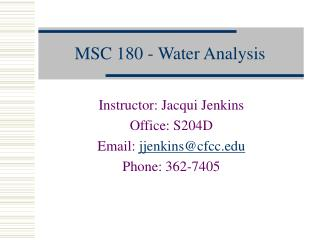DownloadDownload PresentationMSC 180 - Water Analysis

# MSC 180 - Water Analysis

Download Presentation## MSC 180 - Water Analysis

- - - - - - - - - - - - - - - - - - - - - - - - - - - E N D - - - - - - - - - - - - - - - - - - - - - - - - - - -
##### Presentation Transcript

1. MSC 180 - Water Analysis Instructor: Jacqui Jenkins Office: S204D Email: jjenkins@cfcc.edu Phone: 362-7405

2. Laboratory Safety • Safety Requirements

3. Safety Equipment • Eyewash & Shower • First Aid Kit

4. Fire & Fire Safety • Fire Blanket • Fire Extinguisher

5. Safety Equipment • MSDS • Material Safety Data Sheets

6. The Metric System • Decimal system of measurement • Base of 10 • History • France, 1791 • US, Liberia, and Myanmar (Burma) • http://lamar.colostate.edu/~hillger/

7. The Metric System - Prefixes • micro = µ = one-millionth = 10-6 • milli = m = one-thousandth = 10-3 • centi = c = one-hundredth = 10-2 • deci = d = one-tenth = 10-1 • deka = dk = ten times = 101 • hecto = h = hundred times = 102 • kilo = k = thousand times = 103 • mega = M = million times = 106

8. The Metric System • Length • Meter • Weight • Gram • Volume • Liter

9. The Metric System - Length • 1 micrometer (µm) = 0.000001 meter • 1 millimeter (mm) = 0.001 meter • 1 centimeter (cm) = 0.01 meter • 1 decimeter (dm) = 0.1 meter • 1 dekameter (dkm) = 10 meters • 1 hectometer (hm) = 100 meters • 1 kilometer (km) = 1000 meters

10. The Metric System – Weight (Mass) • 1 microgram (µg) = 0.000001 gram • 1 milligram (mg) = 0.001 gram • 1 centigram (cg) = 0.01 gram • 1 decigram (dg) = 0.1 gram • 1 dekagram (dkg) = 10 grams • 1 hectogram (hg) = 100 grams • 1 kilogram (kg) = 1000 grams

11. The Metric System - Volume • 1 microliter (µL) = 0.000001 liter • 1 milliliter (mL) = 0.001 liter • 1 centiliter (cL) = 0.01 liter • 1 deciliter (dL) = 0.1 liter • 1 dekaliter (dkL) = 10 liters • 1 hectoliter (hL) = 100 liters • 1 kiloliter (kL) = 1000 liters

12. 1012 microphones = 1 megaphone 106 bicycles = 2 megacycles 2000 mockingbirds = 2 kilomockingbirds 10 cards = 1 decacards 10¯6 fish = 1 microfiche 454 graham crackers = 1 pound cake 1012 pins = 1 terrapin 10 rations = 1 decoration 10 millipedes = 1 centipede 3 1/3 tridents = 1 decadent 10 monologs = 5 dialogues 2 monograms = 1 diagram 8 nickels = 2 paradigms 2 snake eyes = 1 paradise 2 wharves = 1 paradox 10¯6 phones = 1 microphone 106 phones = 1 megaphone 10¯2 mental = 1 centimental 10¯1 mate = 1 decimate 10¯12 boos = 1 picoboo

13. Conversion • Metric English Equivalents • 1 cm = 0.3937 in. • 1 m = 3.281 ft = 39.37 in. • 1 km = 0.6214 mile • 1 L = 0.2646 gal • 1 kg = 2.204 lbs

14. The Metric System • Temperature • Celsius (°C) • Kelvin (K) • Absolute zero • 0 K • -273.15 °C

15. Conversion • K = °C + 273 • °C = 5/9(°F - 32) • °F = 9/5(°C +32)

16. The Metric System • Density • Mass per unit volume • D = M/V • Mass in grams • Volume in cubic centimeters • g/cm3

17. Lab Procedures • Recording Data/Lab Notebooks • Ink • Never rip out pages • Never erase data; cross out mistakes • Record all information, no matter how trivial • Be specific • Check all calculations • And, obviously, BE NEAT

18. Lab Procedures • Bunsen burners

19. Lab Procedures • Glass Tubing • Cutting • Fire Polishing • Bending • Inserting into stoppers

20. Lab Procedures • Transfer of Liquids • Correct handling of glass stoppers • Pouring liquids from beakers

21. Lab Procedures • Loosening stuck stopcocks and stoppers

22. Lab Procedures • Weighing/Balances • Most important tool in a chemistry lab • Used to determine the mass of an object • Triple-beam balance • Analytical balance

23. Precision Reproducibility Check by repeated measurements Poor precision results from poor techniques Accuracy Correctness Check by using a different method Poor accuracy results from procedural or equipment flaws Lab Procedures

24. Labware • Section 2 of the handbook • These will be displayed for the rest of the week, then returned to their respective places for the rest of the semester • What is it? • What’s it used for?